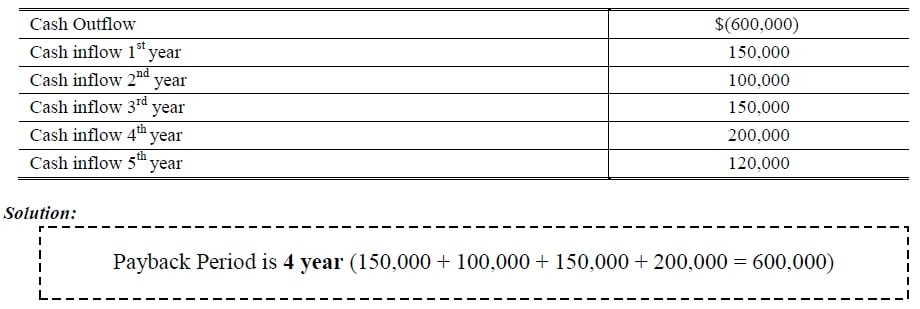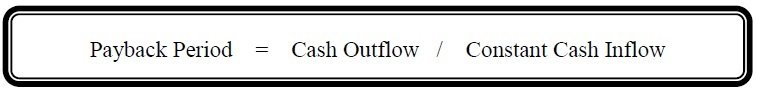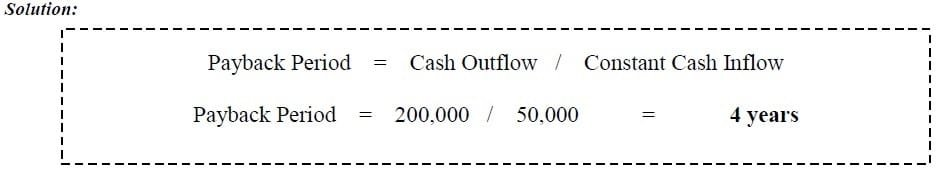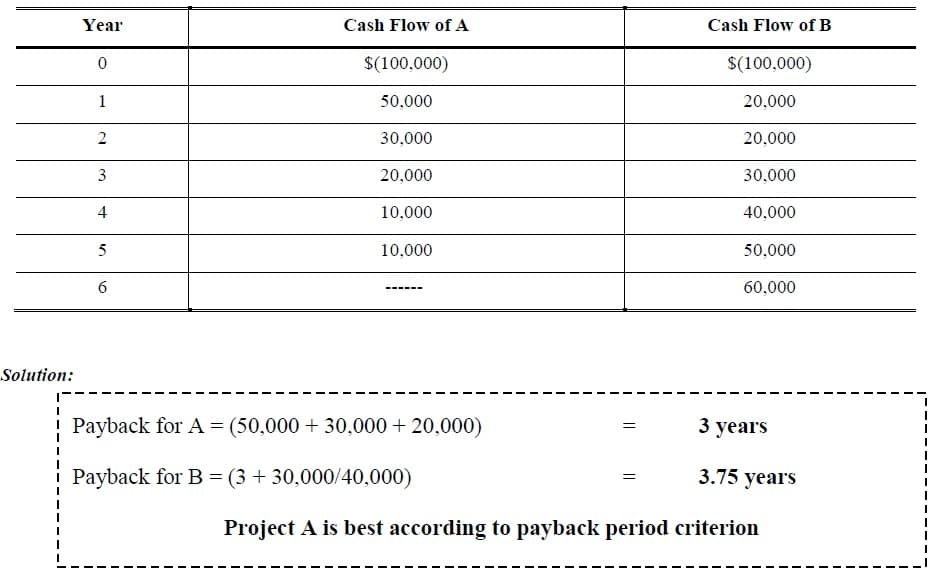Select Page

# Payback Period

Previous Lesson: Capital Budgeting

Next Lesson: Accounting Rate of Return

The Payback Period is the length of time required to recover the initial cash outlay on project. The payback period of the investment tells us the number of years required to cover our initial cash outflow. The major short coming of the payback period is that it fails to consider the cash flows after the payback period.

### Example 1:

Calculate the Payback Period?If Cash inflow is constant than we can apply this equation:### Example 2:

If Cash Outflow is \$200,000 and constant cash inflow will be \$50,000 per year what will be Payback Period?### Example 3:

Which one of the following Investment is best?(Acceptance Criterion: The shorter the Payback Period, the more desirable the project)